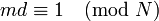# Black-box group algorithm for root computation problem based on power computation problem and order-finding problem

## Summary

Item Value
Problem being solved root computation problem: find the unique$m^{th}$ root of a number where$m$ is relatively prime to the group order.
Problems in terms of which it is being solved power computation problem (can compute any specified integer power of any element of the group)
order-finding problem (can compute the order of the group)
Running time time taken for power computation problem (with exponent at most order of group) + polylog in order of group
If black-box group operations are polylog in order of the group, so is this algorithm.
Variants Instead of the exact order$N$, we simply need some number that is a multiple of the order of the element whose root we are trying to find, and is relatively prime to$m$.
Even if we want something that works for all elements, we could use the exponent of the group instead of the order.

## Idea and outline

Suppose the order of the group is known to be$N$. Use the extended Euclidean algorithm to find a positive integer$d < N$ such that:$md \equiv 1 \pmod N$

Now, to find$h$ such that$h^m = g$ simply compute$h$ as$g^d$.

Note that in fact, the algorithm does not require knowledge of the exact order of the group. It only requires knowledge of a multiple of the order that is still relatively prime to$m$. Moreover, we don't actually need the order of the whole group, we can do with the order of any subgroup of the group containing the element whose root we need to find.